Next: FREQUENCY-STATISTICAL RESOLUTION Up: Resolution Previous: TIME-FREQUENCY RESOLUTION

# TIME-STATISTICAL RESOLUTION

If you flipped a coin 100 times, it is possible that you would get exactly 50 heads'' and 50 tails''. More likely it would be something between 60-40 and 40-60. Typically, how much deviation from 50 would you expect to see? The average (mean) value should be 50, but some other value is almost always obtained from a random sample. The other value is called the sample mean. We would like to know how much difference to expect between the sample mean and the true mean. The average squared difference is called the variance of the sample mean. For a very large sample, the sample mean should be proportionately much closer to the true mean than for a smaller sample. This idea will lead to an uncertainty relation between the probable error in the estimated mean and the size of the sample. Let us be more precise.

The true value'' of the mean could be defined by flipping the coin n times and conceiving of n going to infinity. A more convenient definition of true value'' is that the experiment could be conceived of as having been done separately under identical conditions by an infinite number of people (an ensemble). Such an artifice will enable us to define a time-variable mean for coins which change with time.

The utility of the concept of an ensemble is often subjected to serious attack both from the point of view of the theoretical foundations of statistics and from the point of view of experimentalists applying the techniques of statistics. Nonetheless a great body of geophysical literature uses the artifice of assuming the existence of an unobservable ensemble. The advocates of using ensembles (the Gibbsians) have the advantage over their adversaries (the Bayesians) in that their mathematics is more tractable (and more explainable). So, let us begin!

A conceptual average over the ensemble, called an expectation, is denoted by the symbol E. The index for summation over the ensemble is never shown explicitly; every random variable is presumed to have one. Thus, the true mean at time t may be defined as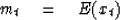(13)
If the mean does not vary with time, we may write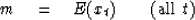(14)

Likewise, we may be interested in a property of xt called its variance which is a measure of variability about the mean defined by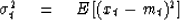(15)
The xt random numbers could be defined in such a way that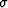or m or both is either time-variable or constant. If both are constant, we have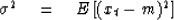(16)
When manipulating algebraic expressions the symbol E behaves like a summation sign, namely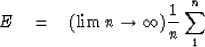(17)
Notice that the summation index is not given, since the sum is over the ensemble, not time.

Now let xt be a time series made up from (identically distributed, independently chosen) random numbers in such a way that m anddo not depend on time. Suppose we have a sample of n points of xt and are trying to determine the value of m. We could make an estimate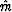of the mean m with the formula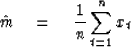(18)
A somewhat more elaborate method of estimating the mean would be to take a weighted average. Let wt define a set of weights normalized so that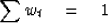(19)
With these weights the more elaborate estimateof the mean is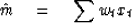(20)
Actually (18) is just a special case of (20) where the weights are wt = 1/n;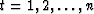.

Our objective in this section is to determine how far the estimated meanis likely to be from the true mean m for a sample of length n. One possible definition of this excursion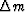is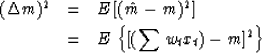(21) (22)
Now utilize the fact that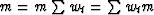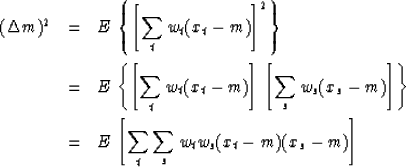(23) (24) (25)
Now the expectation symbol E may be regarded as a summation sign and brought inside the sums on t and s.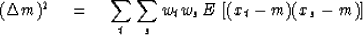(26)
By the randomness of xt and xs the expectation on the right, that is, the sum over the ensemble, gives zero unless s = t. If s = t, then the expectation is the variance defined by (16). Thus we have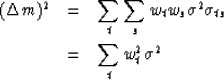(27) (28)
or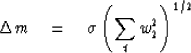(29)
Now let us examine this final result for n weights each of size 1/n. For this case, we get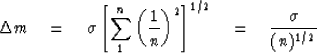(30)
This is the most important property of random numbers which is not intuitively obvious. For a zero mean situation it may be expressed in words:   n random numbers of unit magnitude add up to a magnitude of about the square root of n.''

When one is trying to estimate the mean of a random series which has a time-variable mean, one faces a basic dilemma. If one includes a lot of numbers in the sum to getsmall, then m may be changing while one is trying to measure it. In contrast,measured from a short sample of the series might deviate greatly from the true m (defined by an infinite sum over the ensemble at any point in time). This is the basic dilemma faced by a stockbroker when a client tells him, Since the market fluctuates a lot I'd like you to sell my stock sometime when the price is above the mean selling price.''

If we imagine that a time series is sampled every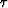seconds and we let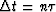denote the length of the sample then (30) may be written as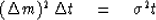(31)
It is clearly desirable to have bothand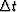as small as possible. If the original random numbers xt were correlated with one another, for example, if xt were an approximation to a continuous function, then the sum of the n numbers would not cancel to root n. This is expressed by the inequality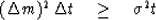(32)
The inequality (32) may be called an uncertainty relation between accuracy and time resolution.

In considering other sets of weights one may take a definition ofwhich is more physically sensible thantimes the number of weights. For example, if the weights wt are given by a sampled gaussian function as shown in Figure 2 thencould be taken as the separation of half-amplitude points, 1/e points, the time span which includes 95 percent of the area, or it could be given many other sensible'' interpretations. Given a little slop in the definition ofand,it is clear that the inequality of (32) is not to be strictly applied.

 4-1 Figure 2 Binomial coefficients tend to the gaussian function. Plotted are the coefficients of Zt in (.5 + .5Z)20.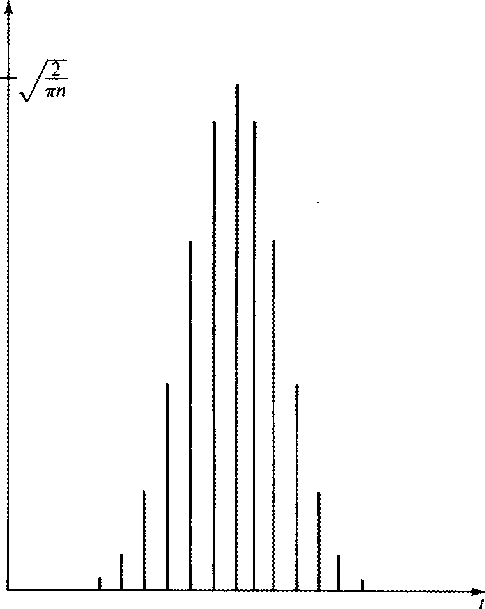Given a sample of a zero mean random time series xt, we may define another series yt by yt = x2t. The problem of estimating the variance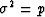of xt is identical to the problem of estimating the mean m of yt. If the sample is short, we may expect an error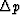in our estimate of the variance. Thus, in a scientific paper one would like to write for the mean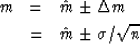(33) (34)
but since the variance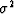often is not known either, it is necessary to use the estimated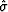, that is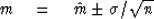(35)

Of course (35) really is not right because we really should add something to indicate additional uncertainty due to error in. This estimated error would again have an error, ad infinitum. To really express the result properly, it is necessary to have a probability density function to calculate all the E(xn) which are required. The probability function can be either estimated from the data or chosen theoretically. In practice, for a reason given in a later section, the gaussian function often occurs. In the exercise it is shown that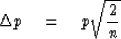(36)
Since, by squaring we have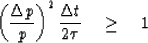(37)
The inequality applies if the random numbers xt are not totally unpredictable random numbers. If xt is an approximation to a continuous function, then it is highly predictable and there will be a lot of slack in the inequality.

Correlation is a concept similar to cosine. A cosine measures the angle between two vectors. It is given by the dot product of the two vectors divided by their magnitudes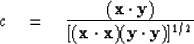Correlation is the same sort of thing, except x and y are scalar random variables, so instead of having a vector subscript their subscript is the implicit ensemble subscript. Correlation is defined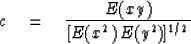In practice one never has an ensemble. There is a practical problem when the ensemble average is simulated by averaging over a sample. The problem arises with small samples and is most dramatically illustrated for a sample with only one element. Then the sample correlation is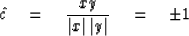regardless of what value the random number x or the random number y should take. In fact, it turns out that the sample correlationwill always scatter away from zero.

No doubt this accounts for many false discoveries''. The topic of bias and variance of coherency estimates is a complicated one, but a rule of thumb seems to be to expect bias and variance ofon the order of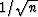for samples of size n.

## EXERCISES:

1. Suppose the mean of a sample of random numbers is estimated by a triangle weighting function, i.e.,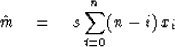Find the scale factor s so that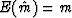. Calculate.Define a reasonable. Examine the uncertainty relation.
2. A random series xt with a possibly time-variable mean may have the mean estimated by the feedback equation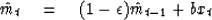(a)
Express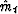as a function of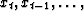and not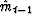.
(b)
What is, the effective averaging time?

(c)
Find the scale factor b so that if mt = m, then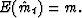(d)
Compute the random error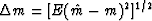[answer goes to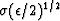asgoes to zero].

(e)
What is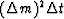in this case?

3. Show that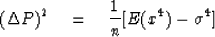4. Define the behavior of an independent zero-mean-tie series xt by defining the probabilities that various amplitudes will be attained. Calculate E(xi), E(x2i),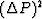.If you have taken a course in probability theory, use a gaussian probability density function for xi. HINT: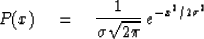and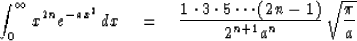Next: FREQUENCY-STATISTICAL RESOLUTION Up: Resolution Previous: TIME-FREQUENCY RESOLUTION
Stanford Exploration Project
10/30/1997